• 常见运算符有那些： 算术运算符：（+，-，，/，%）算术运算符是一些数学最基础的运算符。 关系运算符：（>，<，=，>=，<=，==，!=）这些运算符是用来进行比较的，而最终的比较结果是一个boolean值。 ...
常见的运算符有那些：
算术运算符：（+，-，，/，%）算术运算符是一些数学最基础的运算符。*
关系运算符：（>，<，=，>=，<=，==，!=）这些运算符是用来进行比较的，而最终的比较结果是一个boolean值。
逻辑运算符：与（&&，&）与表示的是两边的条件都要达成，前面的符号是断路与比后面的效率能高一点。
或（||，|）或表示的两边的条件达成一个就成立，前面的符号是断路或比后面的效率也能高一点。
非（！）非表示不成立。
自加和自减运算符：自加：i++,++i
自减：i–，--i     自加和自减加号减号放前面后面的区别是符号放前面优先级特别高，符号放后面优先级特别低。
赋值运算符：（=，+=，-=，=，/=，%=）等号是将右侧的赋值给左侧，后面五个符号的意思是：   a +=20    这个相当于   a = a+20   其他符合算法大致一样，只是变换+，-，，/和%而已。
三目运算符：变量 = 表达式是否成立？第一个值：第二个值      这个的意思是一个变量等于这个表达式，然后这个表达式如果成立则输出第一个值如果不成立则输出第二个值。
程序的控制流程：
顺序：从左到右，从上往下来进行。
选择结构：if语句：
单分支if
if (条件判断){如果条件为真，则执行{}内的代码}
双分支if   else
if(条件判断){如果条件为真，则执行if内的代码}
else{如果这个条件为假，则执行else内的代码}
多分支（三分支）if      else if        else if
if(条件一){条件一成立执行内部代码}
else if(条件二){条件二成立执行内部代码}
else if(条件三){条件三成立执行内部代码}
switch语句：
switch（变量）{
case值1：
//当变量对应值为1时，执行这里的代码
break;
case值2：
//当变量对应值为2时，执行这里的代码
break;
…
default:
//表示剩下的语句
break；
}
break的作用：
在switch语句中终止switch语句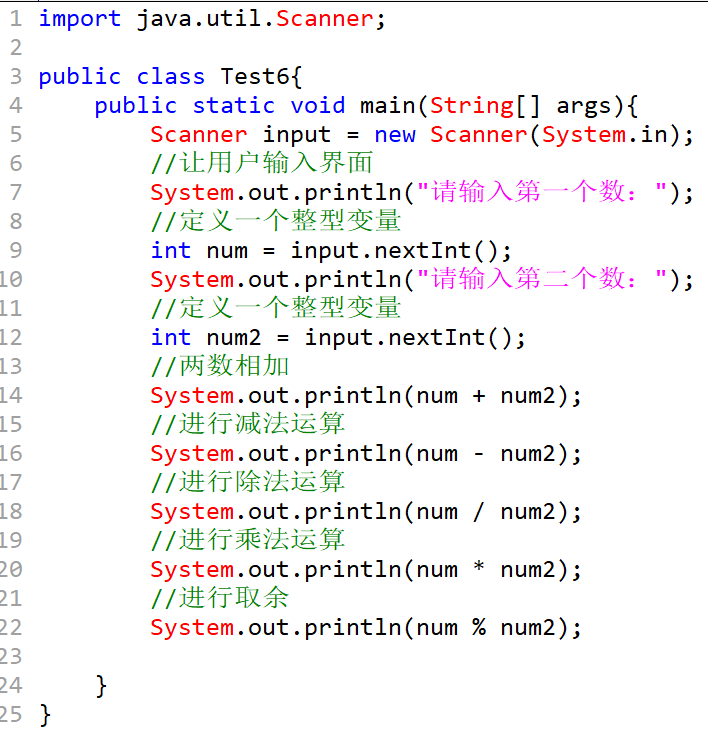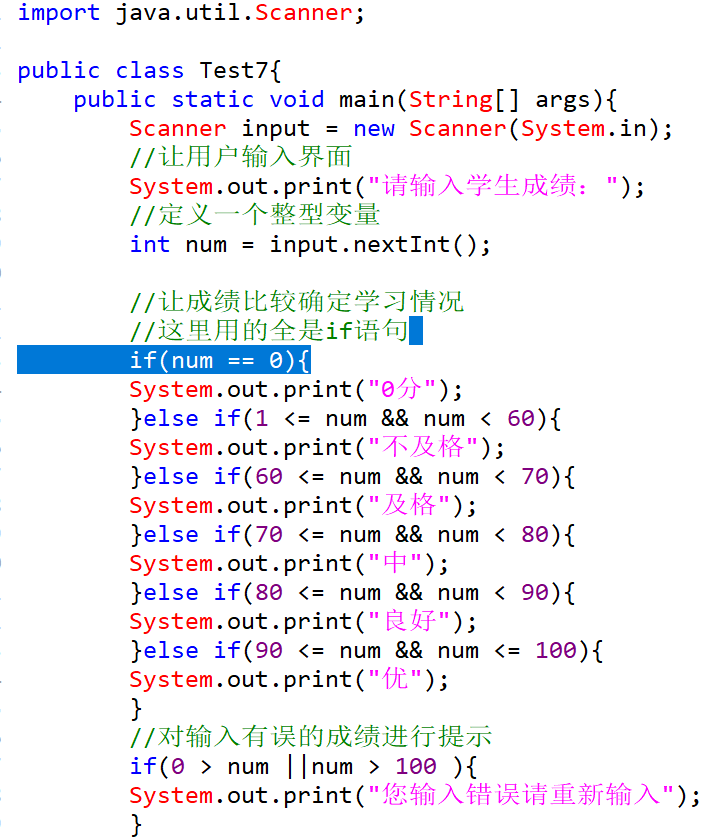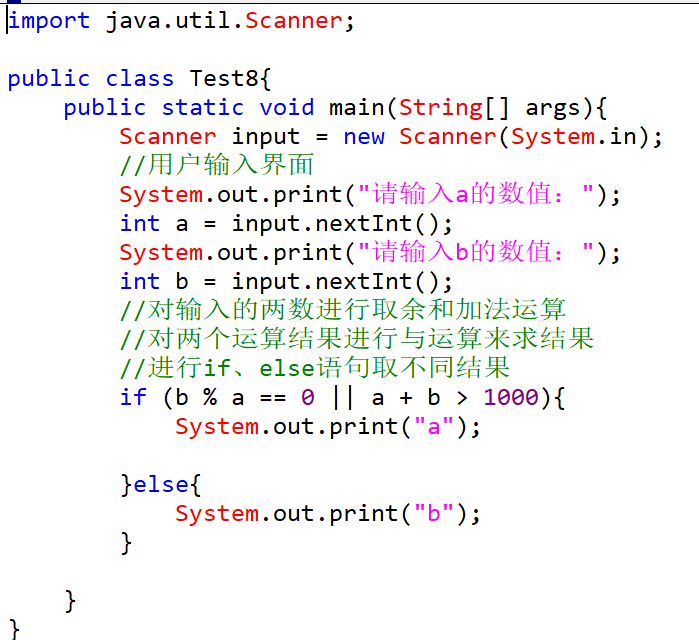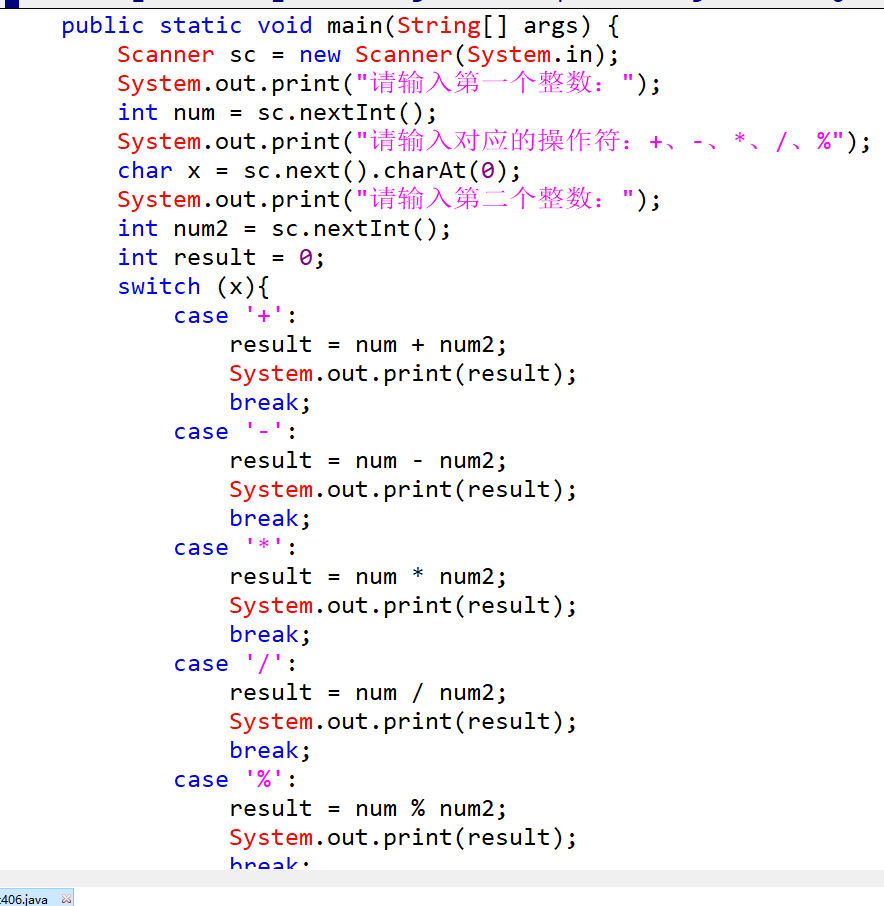展开全文• 赋值运算符用来把右侧的值...=是 Python 中最常见、最基本的赋值运算符，用来将一个表达式的值赋给另一个变量，请看下面的例子： #将字面量（直接量）赋值给变量 n1 = 100 f1 = 47.5 s1 = "http://c.bianche..
赋值运算符用来把右侧的值传递给左侧的变量（或者常量）；可以直接将右侧的值交给左侧的变量，也可以进行某些运算后再交给左侧的变量，比如加减乘除、函数调用、逻辑运算等。Python 中最基本的赋值运算符是等号=；结合其它运算符，=还能扩展出更强大的赋值运算符。

基本赋值运算符

=是 Python 中最常见、最基本的赋值运算符，用来将一个表达式的值赋给另一个变量，请看下面的例子：

#将字面量（直接量）赋值给变量
n1 = 100
f1 = 47.5
s1 = "http://c.biancheng.net/python/"

#将一个变量的值赋给另一个变量
n2 = n1
f2 = f1

#将某些运算的值赋给变量
sum1 = 25 + 46
sum2 = n1 % 6
s2 = str(1234) #将数字转换成字符串
s3 = str(100) + "abc"
连续赋值

Python 中的赋值表达式也是有值的，它的值就是被赋的那个值，或者说是左侧变量的值；如果将赋值表达式的值再赋值给另外一个变量，这就构成了连续赋值。请看下面的例子：

a = b = c = 100

=具有右结合性，我们从右到左分析这个表达式：

c = 100 表示将 100 赋值给 c，所以 c 的值是 100；同时，c = 100 这个子表达式的值也是 100。
b = c = 100 表示将 c = 100 的值赋给 b，因此 b 的值也是 100。
以此类推，a 的值也是 100。

最终结果就是，a、b、c 三个变量的值都是 100。

= 和 ==

= 和 == 是两个不同的运算符，= 用来赋值，而 == 用来判断两边的值是否相等，千万不要混淆。

扩展后的赋值运算符

=还可与其他运算符（包括算术运算符、位运算符和逻辑运算符）相结合，扩展成为功能更加强大的赋值运算符，如表 1 所示。扩展后的赋值运算符将使得赋值表达式的书写更加优雅和方便。

表 1 Python 扩展赋值运算符
运算符
说 明
用法举例
等价形式
=
最基本的赋值运算
x = y
x = y
+=
加赋值
x += y
x = x + y
-=
减赋值
x -= y
x = x - y
*=
乘赋值
x *= y
x = x * y
/=
除赋值
x /= y
x = x / y
%=
取余数赋值
x %= y
x = x % y
**=
幂赋值
x **= y
x = x ** y
//=
取整数赋值
x //= y
x = x // y
&=
按位与赋值
x &= y
x = x & y
|=
按位或赋值
x |= y
x = x | y
^=
按位异或赋值
x ^= y
x = x ^ y
<<=
左移赋值
x <<= y
x = x << y，这里的 y 指的是左移的位数
>>=
右移赋值
x >>= y
x = x >> y，这里的 y 指的是右移的位数

这里举个简单的例子：

n1 = 100
f1 = 25.5

n1 -= 80 #等价于 n1=n1-80
f1 *= n1 - 10 #等价于 f1=f1*( n1 - 10 )

print("n1=%d" % n1)
print("f1=%.2f" % f1)
运行结果为：
n1=20
f1=255.00
通常情况下，只要能使用扩展后的赋值运算符，都推荐使用这种赋值运算符。
但是请注意，这种赋值运算符只能针对已经存在的变量赋值，因为赋值过程中需要变量本身参与运算，如果变量没有提前定义，它的值就是未知的，无法参与运算。例如，下面的写法就是错误的：

n += 10

该表达式等价于 n = n + 10，n 没有提前定义，所以它不能参与加法运算。

展开全文• 赋值运算符的作用是将一个值赋给一个变量，最常见赋值运算符是“=”。还可以由“=”赋值运算符和其他一些运算符组合产生一些新的赋值运算符，例如，“+=”，“*=”等。 =：将一个值或表达式的结构赋给变量，...

赋值运算符的作用是将一个值赋给一个变量，最常见的赋值运算符是“=”。还可以由“=”赋值运算符和其他一些运算符组合产生一些新的赋值运算符，例如，“+=”，“*=”等。

=：将一个值或表达式的结构赋给变量，例如，x=2。
+=：将变量与所赋的值相加后的结果再赋给该变量，例如，x+=3 等价于 x=x+3。
-=：将变量与所赋的值相减后的结果再赋给该变量，例如，x-=3 等价于 x=x-3。
*=：将变量与所赋的值相乘后的结果再赋给该变量，例如，x*=3 等价于 x=x*3。
/=：将变量与所赋的值相除后的结果再赋给该变量，例如，x/=3 等价于 x=x/3。
%=：将变量与所赋的值求模后的结果再赋给该变量，例如，x%=3 等价于 x=x%3。

转载于:https://www.cnblogs.com/kojya/archive/2013/01/02/2842333.html
展开全文• 算术运算符: 用来处理四则运算的符号 加号 在操作数值、字符、字符串时其结果是不同的，当两个字符相加得到的是ASCII码表值 当两个字符串相加时表示将两个字符串连接在一起，从而组成新的字符串除号 整数在使用...


算术运算符:

用来处理四则运算的符号加号
在操作数值、字符、字符串时其结果是不同的，当两个字符相加得到的是ASCII码表值
当两个字符串相加时表示将两个字符串连接在一起，从而组成新的字符串除号
整数在使用除号操作时，得到的结果仍为整数（小数部分忽略）
当整数除以0的时候，会引发算术异常取模(求余数)
模数的符号忽略不计，结果的正负取决于被模数.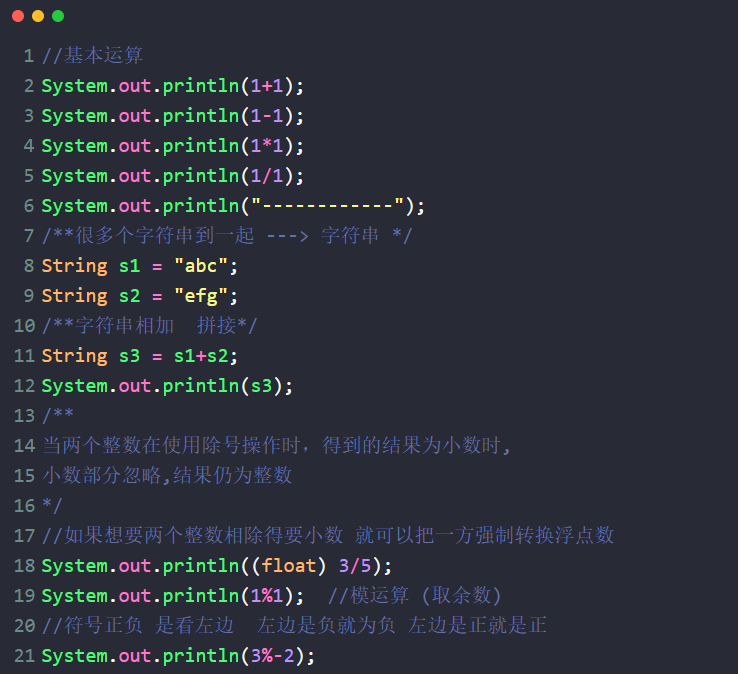自增++与自--
++: 表示当前操作变量自己累加1.
--:   表示当前操作变量自己减去1.
前置（++result）:   表示对result加1之后的结果进行运算
后置（result++）:   表示对result变量加1之前的值（原始值）进行运算。

赋值运算符:

就是将符号右边的值，赋给左边的变量。示例: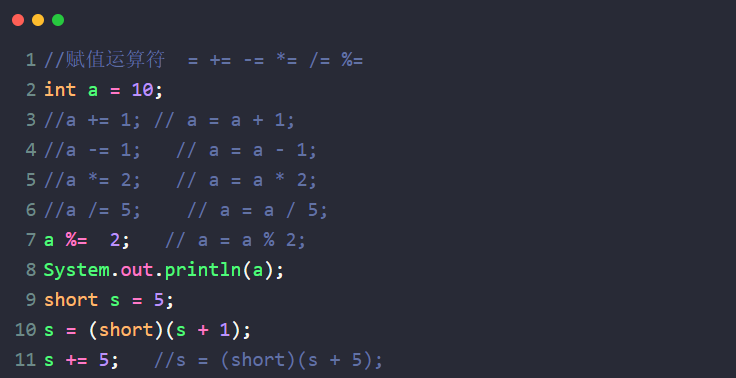比较运算符:用于比较两个变量或常量之间的关系，比较运算符的结果是boolean类型
其操作格式为:boolean  result = 表达式A   比较运算符   表达式B;
比较运算  ==   !=  >  <  >=  <=
比较运算符的结果是一个布尔类型boolean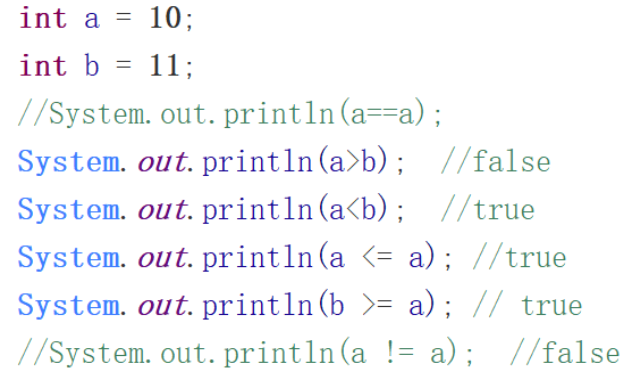逻辑运算符:

是用来连接两个布尔类型结果的运算符，运算结果都是布尔值 true 或者 false
* 所有的逻辑运算符结果都为布尔类型
* 与（&）
* 或（|）
* 非（！）
* 异或（^）
* 短路与(&&)
* 短路或(||)

示例:

与运算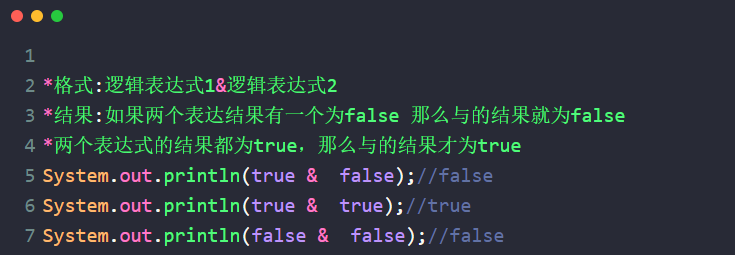或运算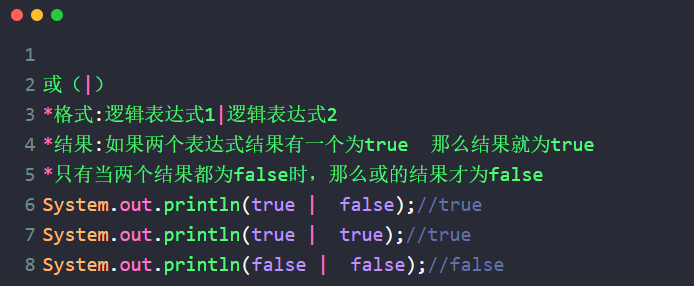非运算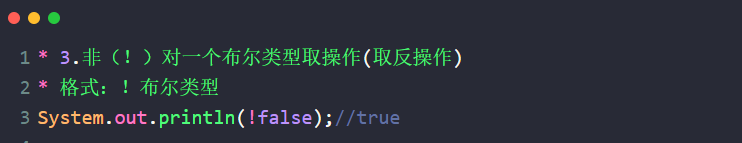异或操作: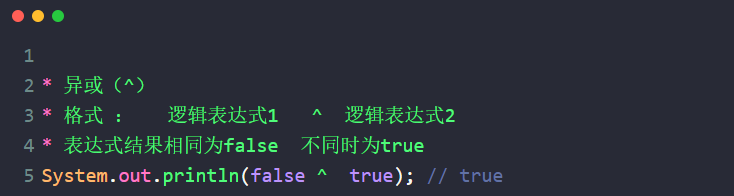短路与(&&)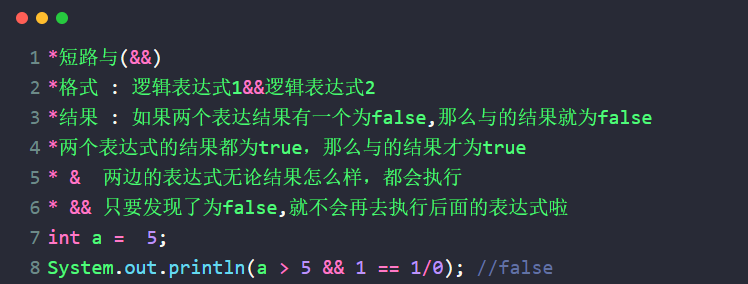短路或(||)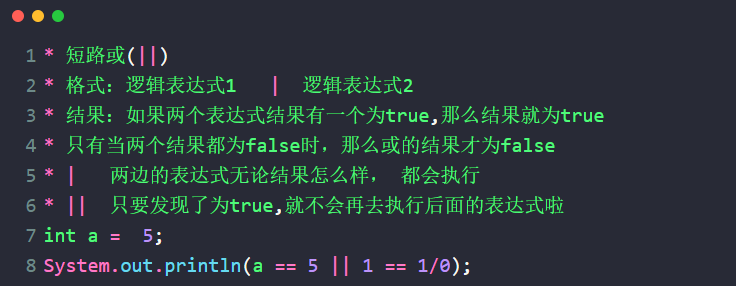详细视频讲解:点击查看


展开全文java
• Java中的赋值运算符 ... 常见五种复合赋值运算符： += -= *= /= %= /*以 %= 为例*/ public class Demo{ public static void main(String[] args){ int e = 120; e %= 7; /*相当于 e = e % 7 *java
• 赋值运算符常见形式： 变量 = 表达式; a = 1+1; //先计算表达式 1+1，然后将结果值赋给变量 a 变量 = 字面量; a= 2; //将数值 2 直接赋值给变量 a 变量 = 变量; a= b; //将变量 b 的值赋值给变量 a 示例代码如...Java语言基础
• 常见赋值运算符就是等号(=)，将右侧的值赋给左侧的变量（表达式x=y表示将y赋值给x） x += y // 等同于 x = x + y 还有其他更多的复合赋值运算 x -= y 等同于 x = x - y x *= y 等同于 x = x * y x...
• 赋值运算符简单的赋值运算符由等号（=）实现，只是把等号右边的值赋予等号...这些赋值运算符是下列这些常见情况的缩写形式：var iNum = 10;iNum = iNum + 10；可以用一个复合赋值运算符改写第二行代码：var iNum = 10;
• 赋值运算符 比较运算符 逻辑运算符 位运算符 三元运算符 注意 常见的运算符 要会使用 用多了就记住了 一个字 敲 赋值运算符 符号：= 扩展赋值运算符：+=、-=、*=、/=、%= 赋值运算符 和我们生活中的理解不一样 我们...java
• 赋值运算符 简单的赋值运算符由等号（=）实现，只是把等号...这些赋值运算符是下列这些常见情况的缩写形式： var iNum = 10; iNum = iNum + 10； 可以用一个复合赋值运算符改写第二行代码： var iNum =...
• 赋值运算符 package day06; public class OperDemo2 { public static void main(String[] args) { /* * 1：赋值运算符： * 1.1)简单赋值运算符：= * 2.2）扩展赋值运算符：+=，-=，*=，/=,%= * ---...
• 赋值运算符用来把右侧的值...=是 Python 中最常见、最基本的赋值运算符，用来将一个表达式的值赋给另一个变量，请看下面的例子： #将字面量（直接量）赋值给变量 n1 = 100 f1 = 47.5 s1 = "http://www.baidu.com/pytho
• 四则运算当中的加号“+”有常见的三种用法 练习：四则运算当中的加号“+”练习 自增自减运算符 练习：自增自减运算符练习 赋值运算符 列：练习赋值运算符 比较运算符 列：练习比较运算符 逻辑运算符 练习：...
• 赋值运算符的种类 基本赋值运算符：就是一个等号“=”，...举例说明赋值运算符常见用法 代码实现 /* 赋值运算符分为： 基本赋值运算符：就是一个等号“=”，代表将右侧的数据交给左侧的变量。例如：int a...
• 2、赋值运算符：=、 +=、 -=、 *=、 /=、 %= 3、条件运算符：==、===、>、>=、<、<=、!=、&&(而且)、||(或者)、!(否) 需求 上一篇张介绍了JavaScript的加法运算，那么下面...
• 赋值运算符 用于将表达式的赋值给变量 比较运算符 用于表达式的比较，并返回一个真值或假值 比较运算符 用于根据表达式的返回值返回真值或假值 3.1算术运算符 作用：用于处理四则运算 算术运算符包括以下符号...c++
• 要求：写出一个String类的赋值运算符函数 注意事项： (1)返回值的类型需声明为该类型的引用，并在函数结束前返回实例自身的引用（即*this），因为只有返回一个引用，才可以允许连续赋值。 (2)传入参数必须为常量...string类 C++
• 赋值运算符用来把右侧的值...是 Python 中最常见、最基本的赋值运算符，用来将一个表达式的值赋给另一个变量，请看下面的例子： #将字面量（直接量）赋值给变量 n1 = 100 f1 = 47.5 s1 = "http://c.biancheng.net/pypython
• 主要介绍：this指针，赋值运算符函数，赋值运算符函数的使用c++ 面向对象编程
• 赋值运算符 比较运算符 逻辑运算符 位运算符 三元运算符 注意 常见的运算符 要会使用 用多了就记住了 一个字 敲 自增 前++ 后++ 前-- 后– 变量自增1 下面用代码进行显示下 前++ 和 后++ 的区别 int a1 = 10; ...java
• 赋值运算符：= 关系运算符：>，<，>=，<=，==，!=，instanceof 逻辑运算符：&&，||，!（与或非） 位运算符：&，|，^，~，>>，<<，>>>（了解！！） 条件运算符：？： ...java 字符串
• Python赋值运算符（入门必读） ...=是 Python 中最常见、最基本的赋值运算符，用来将一个表达式的值赋给另一个变量，请看下面的例子： #将字面量（直接量）赋值给变量 n1 = 100 f1 = 47.5 s1 = "httpython
• 1、简介 C++类中，通常必须要写的三个方法，一些资料中称为big three 拷贝构造，如： String(const String& str); 赋值运算符重载，如： ...这三个函数共通之处，都是为了...下面以赋值运算符的重载为例，简单c++ 指针
• 赋值运算符包括： = += -= *= /= %=class Operate2
• 本篇文章给大家带来的内容是介绍Python中什么是算术运算符、赋值运算符和复合运算符。一定的参考价值，需要的朋友可以参考一下，希望对你们有所助。算术运算符常见的算术运算符 :+ 加法运算符print(1 + 2); //...
• 作者：Joe Seifi 译者：前端小智 ... 点赞再看，微信搜索 【大迁世界】 关注这个没有大厂背景，但有着一股向上积极心态人。... 大家都说简历没项目写，我就帮大家找了一...逻辑赋值是对现有数学和二进制逻辑运算符的扩展。.
• Java提供特殊的算术赋值运算符，该运算符可用来将算术运算符与赋值结合起来。你可能知道，像下列这样的语句在编程中是很常见的： a = a +4; 在Java中，你可将该语句重写如下： a += 4; 该语句使用“+=”进行赋值操作...java 编程语言
• 5. 了解 C++ 默默编写并调用了...但实际上，C++ 会生成这个类的默认构造函数、拷贝构造函数、析构函数、赋值运算符，如下： class Empty { public: Empty() { ... } // 默认构造函数 Empty(const Empty&a...构造函数 C++
• 什么是拷贝赋值运算符 拷贝赋值运算符就是上一章拷贝初始化中提到的“=”，即类与类之间的赋值操作依托赋值运算符进行，此处要注意区别下初始化与赋值的概念。 //person是用户自定义类类型 person p1=p2;//此处为...c++ 编程语言 指针...